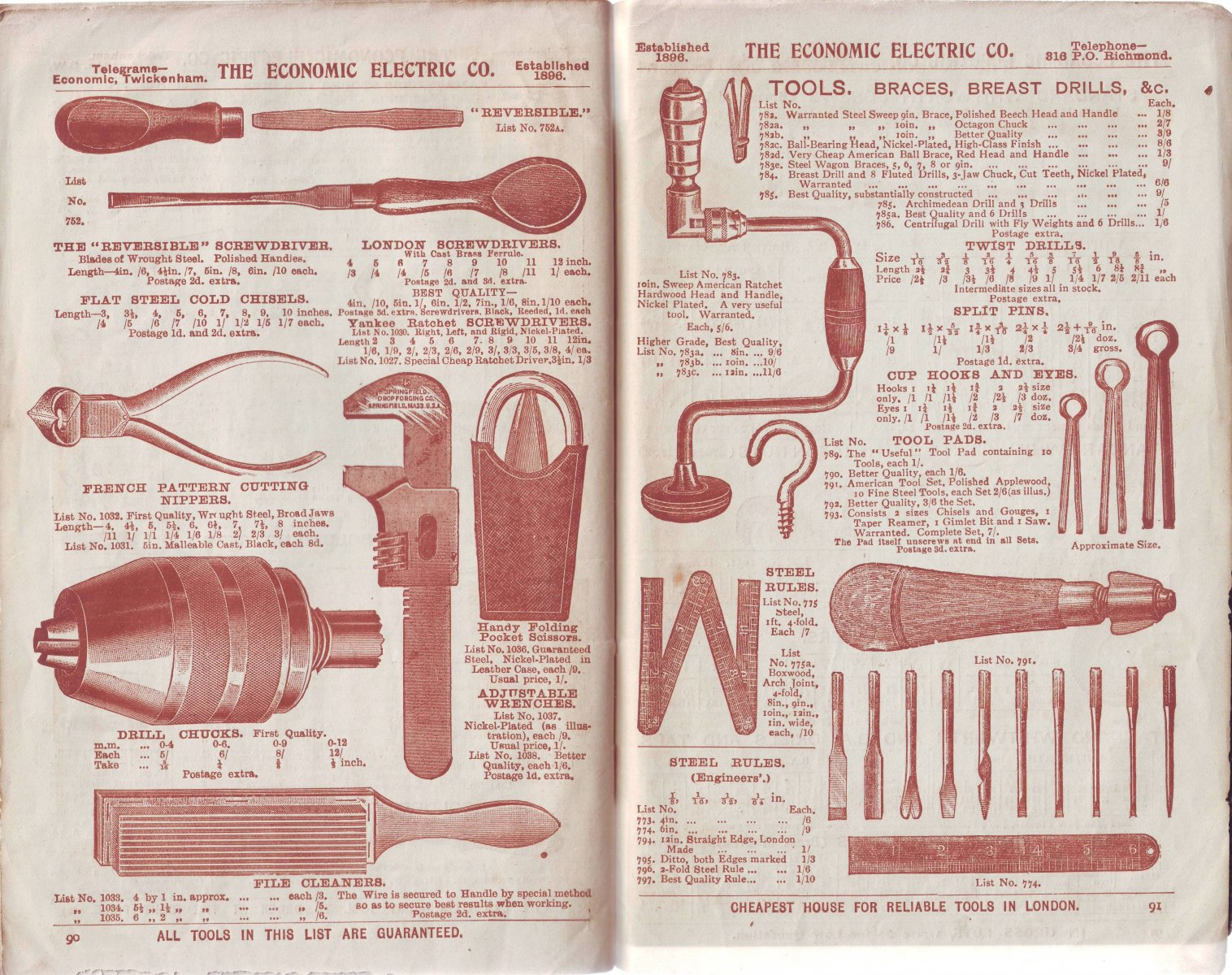Maker's Name:
The Economic Electric Co., Twickenham, London, S.W.
England
Dimensions:
21 × 14 × 0.5 cm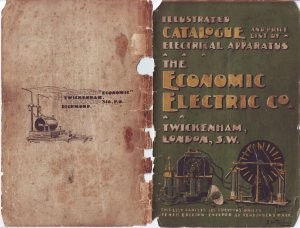Tenth Edition, circa 1905?

Mail order catalogue for all sorts of electrical equipment including bells, telephones, motors, generators, xray, wireless telegraphy, batteries, lamps, medical devices, and electroplating equipment.

A few sample pages show up at the bottom of this page.

All the pages are available below as .jpg files for 400dpi scans. The first ones in the list  are the index from the inside covers.

For browsing, two reduced size .pdf files (8 MB, for Acrobat 6.0 and later) are also available.COVERSIndex 1 and 2index 3p2 and 3p4 and 5p6 and 7p8 and 9p10 and 11p12 and 13p14 and 15p16 and 17p18 and 19p20 and 21p22 and 23p24 and 25p26 and 27p28 and 29p30 and 31p32 and 33p34 and 35p36 and 37p38 and 39p40 and 41p42 and 43p44 and 45p46 and 47p48 and 49p50 and 51p52 and 53p54 and 55p56 and 57p58 and 59p60 and 61p62 and 63p64 and 65p66 and 67p68 and 69p70 and 71p72 and 73p74 and 75p76 and 77p78 and 79p80 and 81p82 and 83p84 and 85p86 and 87p88 and 89p90 and 91p92 and 93p94 and 95p96 and 97Index and pages 2-49Pages 50-97
•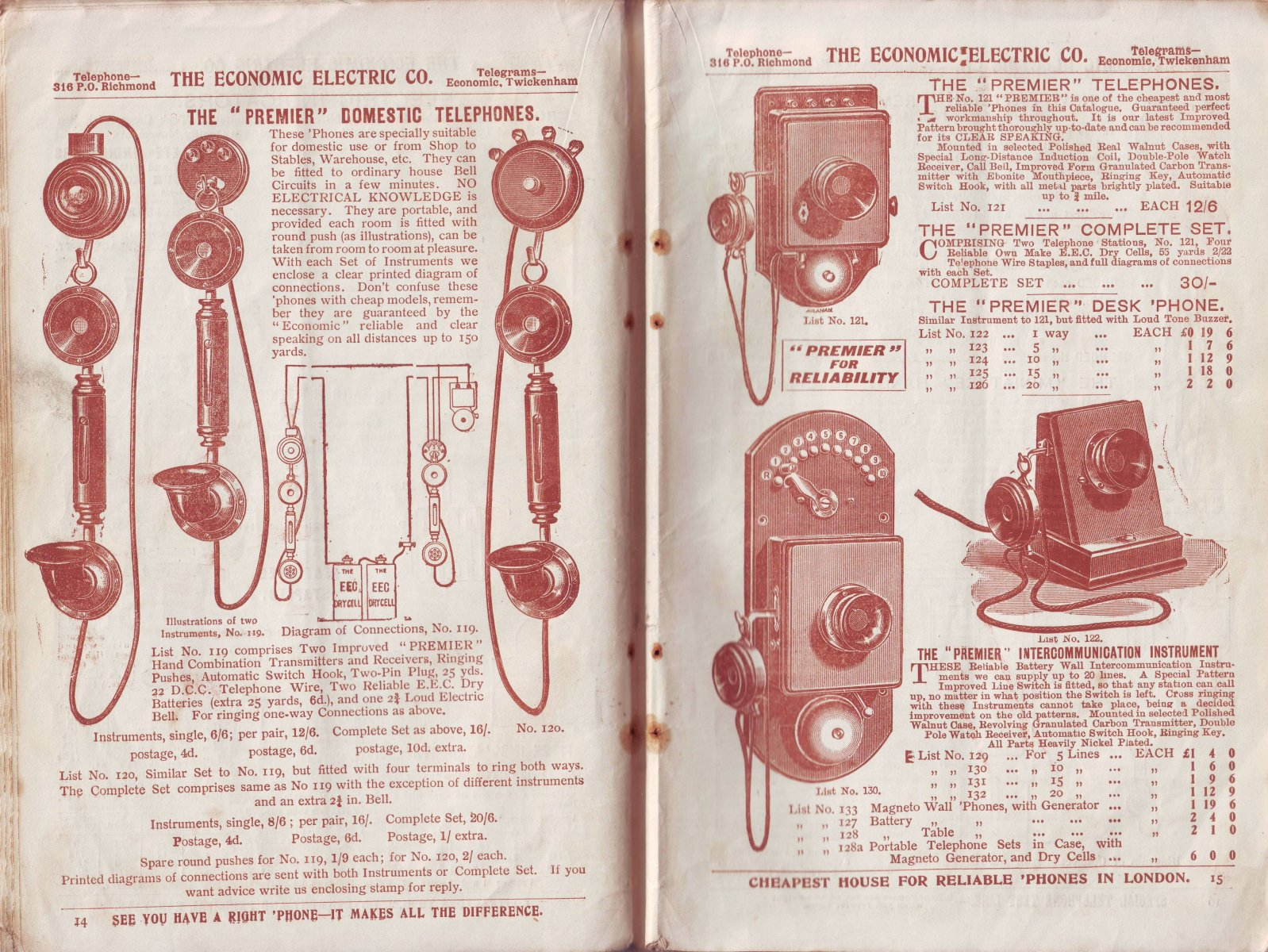p14 and 15
•p18 and 19
•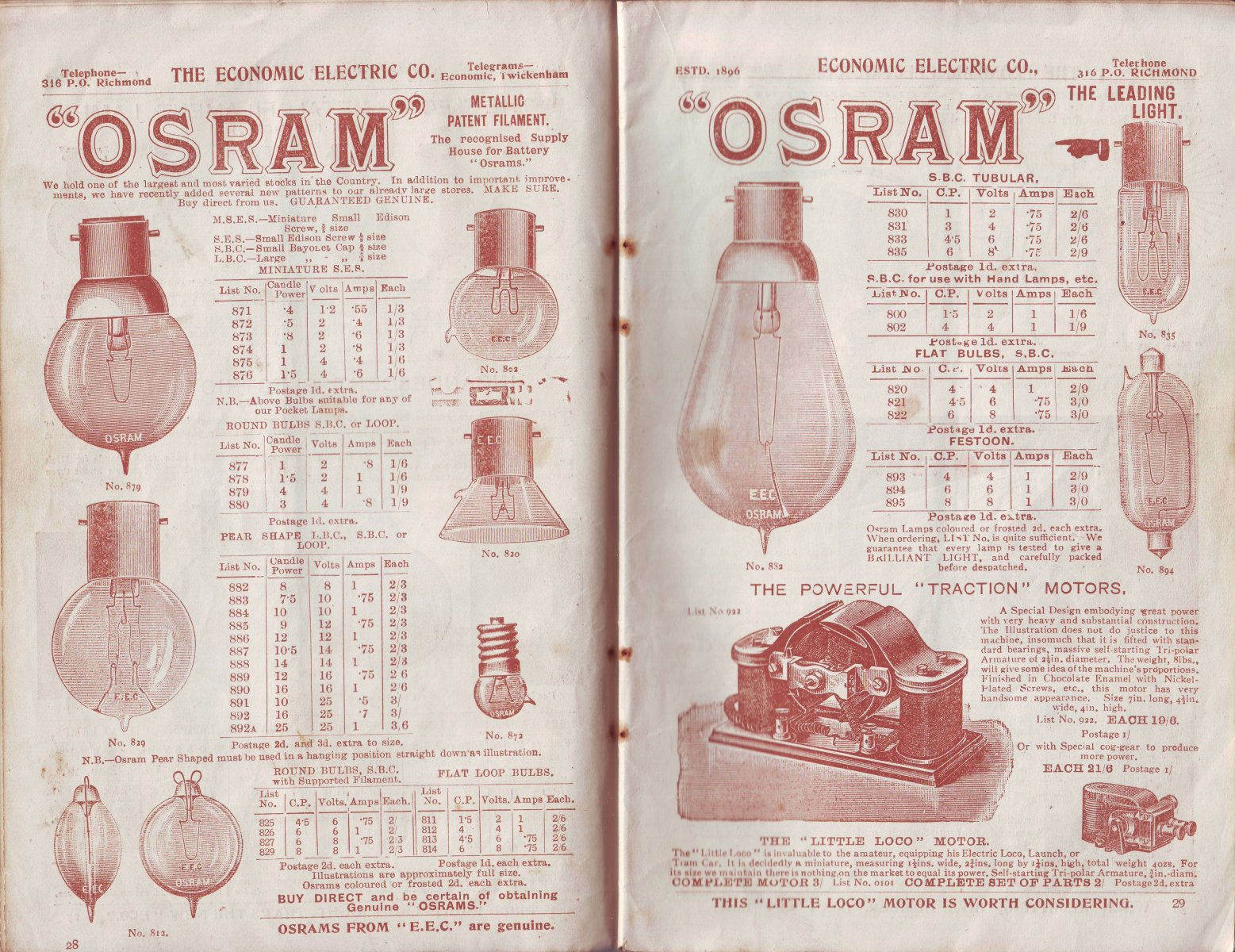p28 and 29
•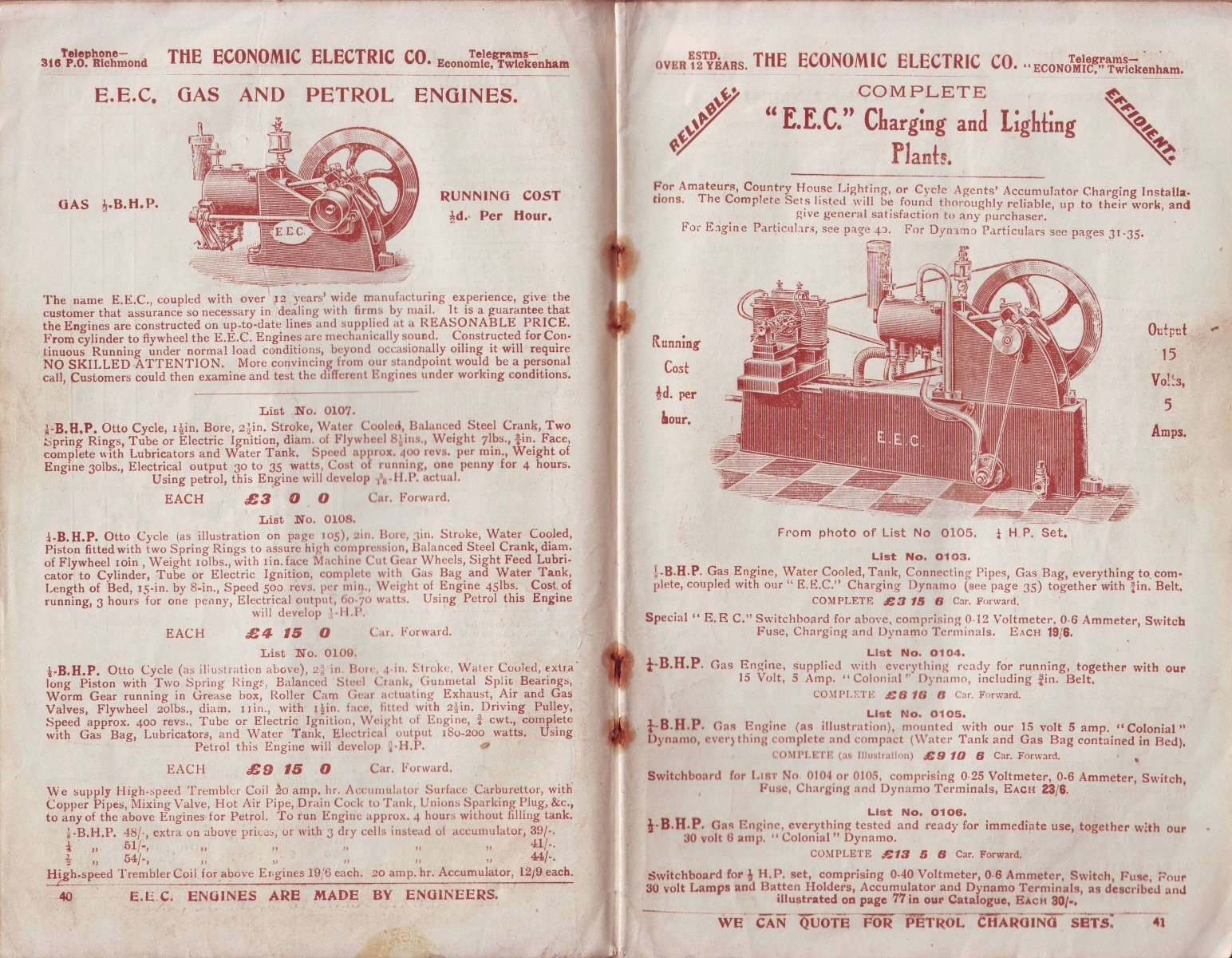p40 and 41
•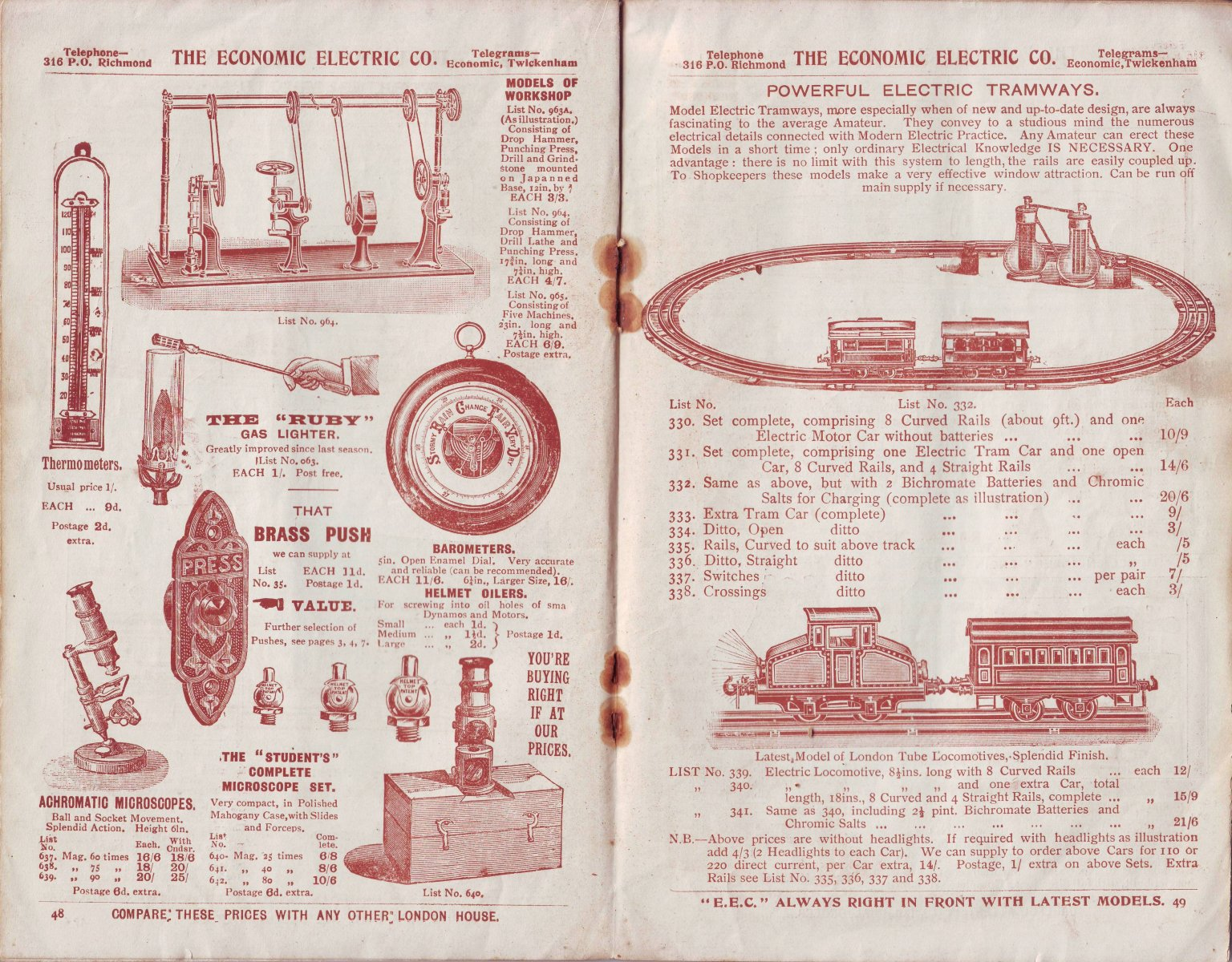p48 and 49
•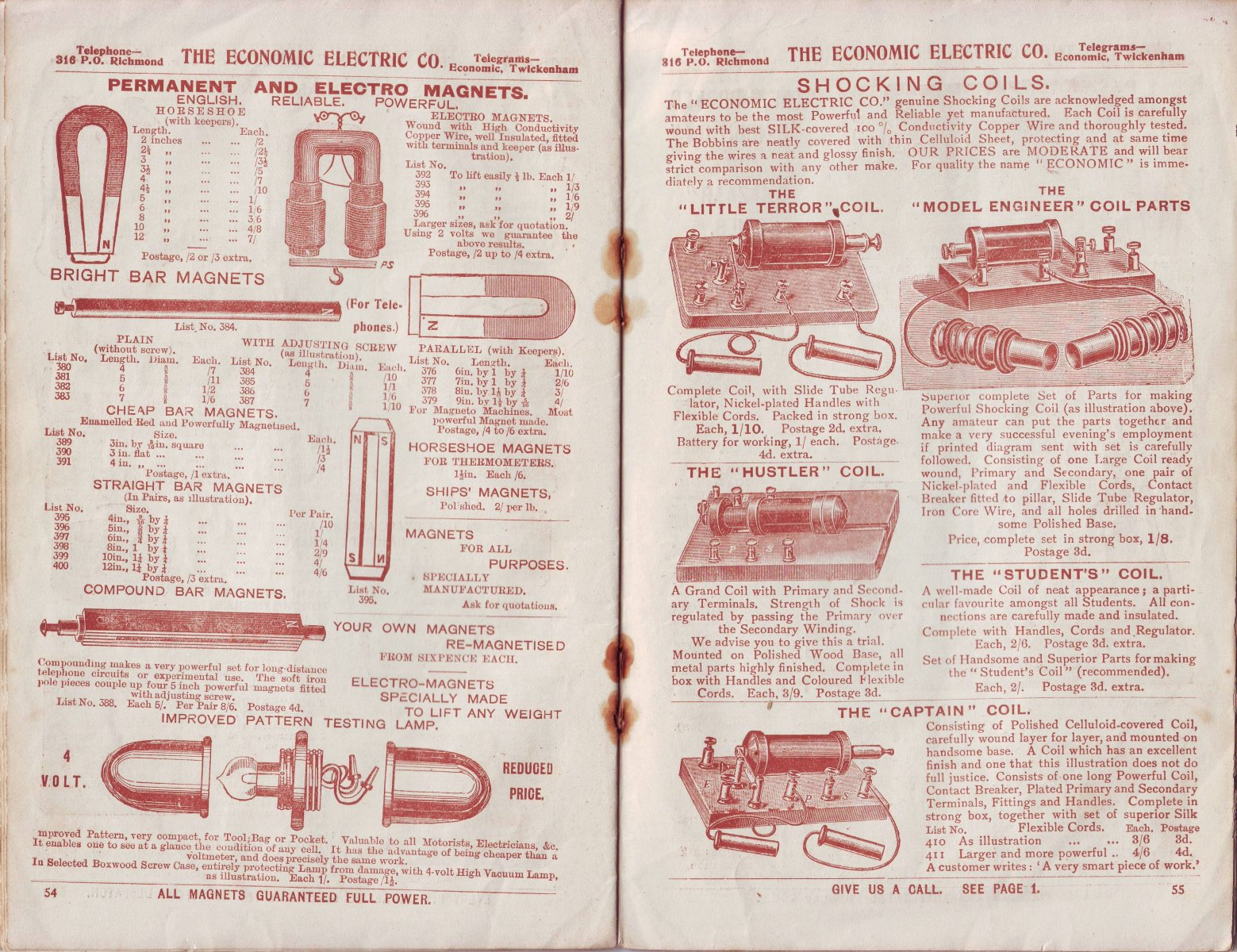p54 and 55
•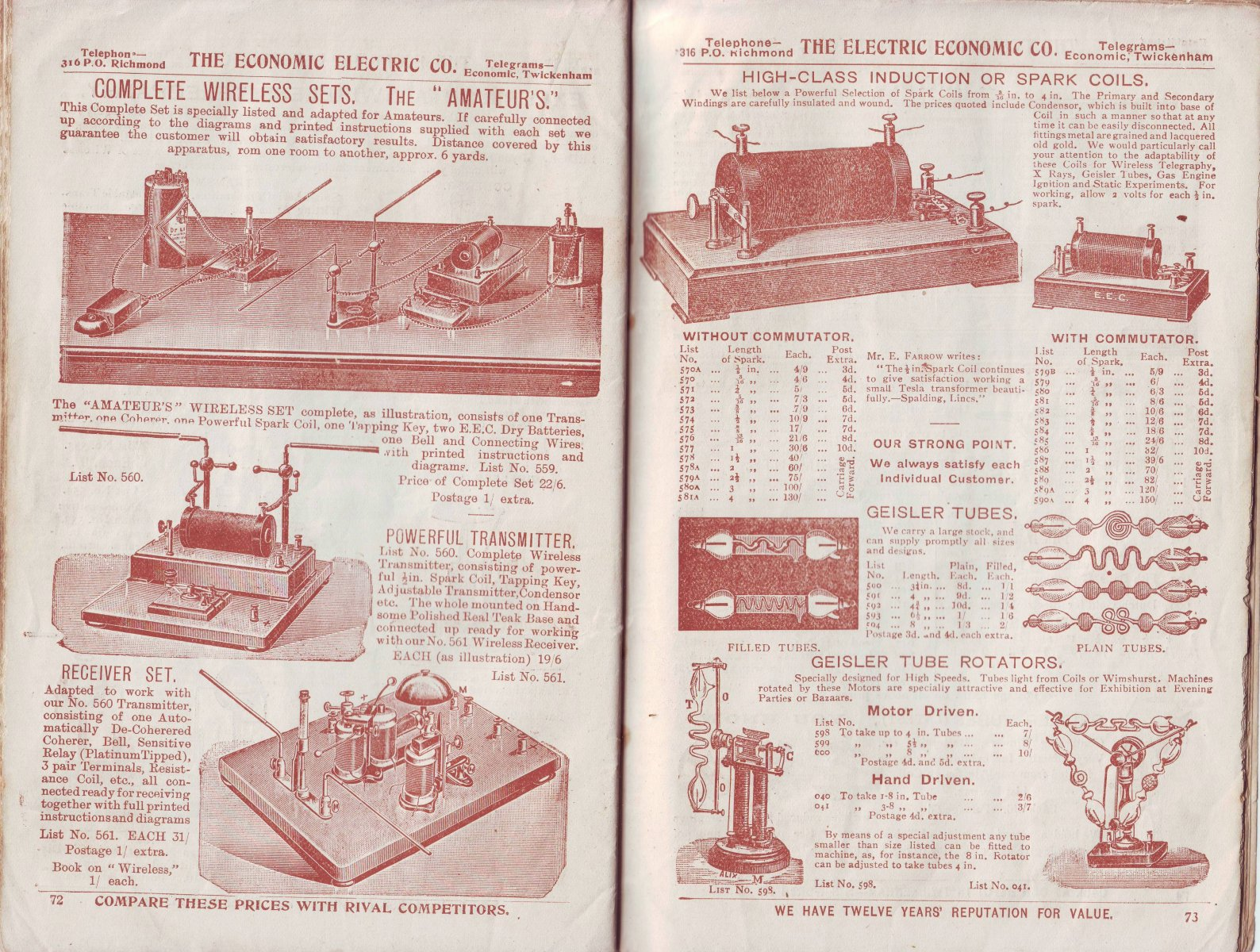p72 and 73
•